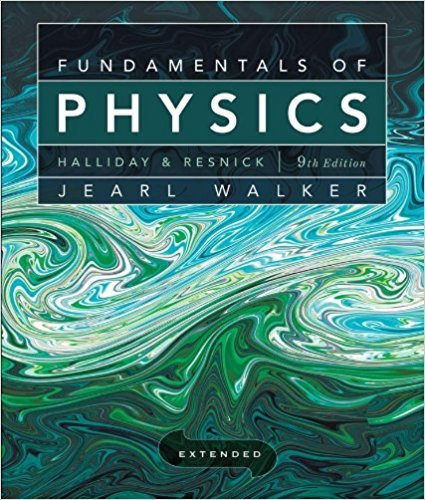×
Log in to StudySoup
Get Full Access to Fundamentals Of Physics Extended - 9 Edition - Chapter 3 - Problem 4
Join StudySoup for FREE
Get Full Access to Fundamentals Of Physics Extended - 9 Edition - Chapter 3 - Problem 4

Already have an account? Login here
×
Reset your password

# Equation 3-2 shows that the addition of two vectors a andISBN: 9780470469088 189

## Solution for problem 4 Chapter 3

Fundamentals of Physics Extended | 9th Edition

• Textbook Solutions
• 2901 Step-by-step solutions solved by professors and subject experts
• Get 24/7 help from StudySoup virtual teaching assistantsFundamentals of Physics Extended | 9th Edition

4 5 1 273 Reviews
19
1
Problem 4

Equation 3-2 shows that the addition of two vectors a and b is commutative. Does that mean subtraction is commutative, so that a - b = b -a?

Step-by-Step Solution:
Step 1 of 3

h t g Week3Notes n • AverageVelocity e o v=Δx/Δt • AverageSpeed L o PathLength/Δt h t - a -- P -- Δ - - Example#1:BIKETRIP North5blocksin5minutes East3blocksin6minutes South5blocksin10minutes Findthetotalaveragespeedandtotalaveragevelocity. PathLength=5+3+5=13

Step 2 of 3

Step 3 of 3

##### ISBN: 9780470469088

This full solution covers the following key subjects: . This expansive textbook survival guide covers 37 chapters, and 3413 solutions. The answer to “Equation 3-2 shows that the addition of two vectors a and b is commutative. Does that mean subtraction is commutative, so that a - b = b -a?” is broken down into a number of easy to follow steps, and 28 words. The full step-by-step solution to problem: 4 from chapter: 3 was answered by , our top Physics solution expert on 12/27/17, 08:15PM. This textbook survival guide was created for the textbook: Fundamentals of Physics Extended, edition: 9. Since the solution to 4 from 3 chapter was answered, more than 308 students have viewed the full step-by-step answer. Fundamentals of Physics Extended was written by and is associated to the ISBN: 9780470469088.

Unlock Textbook Solution

Enter your email below to unlock your verified solution to:

Equation 3-2 shows that the addition of two vectors a and